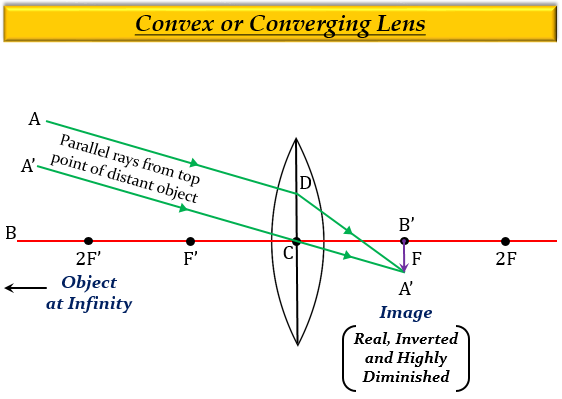# If an object is at a considerable distance (or infinity) in front of a convex lens, where is the image formed?

If an object is at a considerable distance (or infinity) in front of a convex lens, then the image will be formed at the focus $(F)$.

Explanation

When an object is at a considerable distance (or infinity) in front of a convex lens, then the image formed is real, inverted, much smaller than the object (or highly diminished), and at the focus $(F)$ on the other side of the lens.Updated on: 10-Oct-2022

230 Views